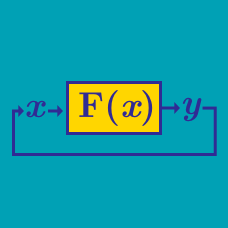Computer Science

# Variables and Scope

Suppose that one day you and a group of friends decide to come up with a brand new programming language, with syntax similar to Java's. After it is finished you decide to test it out with a program that prints two integers on the same line as shown below:

  1 2 3 4 5 6 7 8 9 10 11 12 13 14 15 16 17 18 19 20 int x; def int main() { x = 7; f(); g(); } def void f() { int x = 35; h(); } def void g() { int x = 29; h(); } def void h() { printf("%d\n",x); } 

You and your friends still have not yet agreed on whether the programming language should follow either static scoping or dynamic scoping.

If if follows static scoping it will print out

 1 a b 

where $a$ and $b$ are integers.

If it follows dynamic scoping, it will print out

 1 c d 

where $c$ and $d$ are integers.

Find the value of $a + b + c + d$

The prime factors of a number $n$ are all the prime numbers that divide it. For example the prime factors of $2015$ are 13, 31, and 5 ($2015 = 5 \times 13 \times 31$). The following Python function finds the largest prime factor of a number.

  1 2 3 4 5 6 7 8 9 10 def largest_pf(n): for divisor_1 in range(1, n + 1): for divisor_2 in range(2, divisor_1 + 1): is_prime = True if divisor_1 % divisor_2 == 0: is_prime = False break if is_prime: max_divisor = divisor_1 return max_divisor 

The program works well for most inputs but it contains a variable whose scope is not properly defined. Identify the variable.

  1 2 3 4 5 6 7 8 9 10 11 12 01 int x = 7; 02 namespace MyNamespace 03 { 04 int x = 11; 05 void f() 06 { 07 int x = 42; 08 std::cout << x << std::endl; 09 std::cout << MyNamespace::x << std::endl; 10 std::cout << ::x << std::endl; 11 } 12 } 

Consider the above algorithm, in c++ the $::$ notation is used to refer back to the the variable defined in the global namespace. Let $l_{1},l_{2}$ and $l_{3}$ be the output results of line $8$, $9$ and $10$, respectively then what is the value of

$(l_{1}+l_{2})-l_{3}?$

  1 2 3 4 5 6 7 8 9 10 11 12 13 14 15 16 def leave(): code... return 11 find = 12 def bad(): for code... code... find = 20 def leave(): return find + 1 return leave() print find + bad() + leave() 

Consider the fragment of theoretical python code. Predict what will be outputted when it is run once.

Side note: This is an example of very poor function and variable naming. Proper naming in programming is one of the keys to writing good code.

×